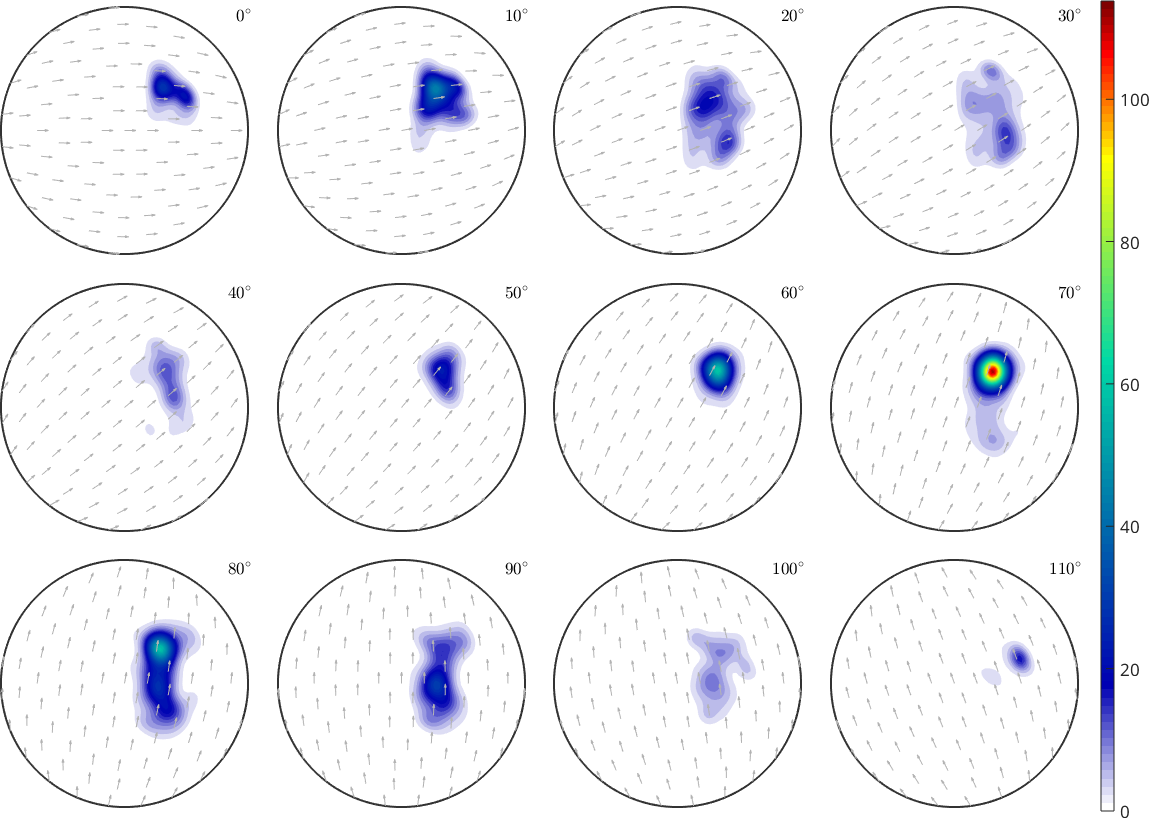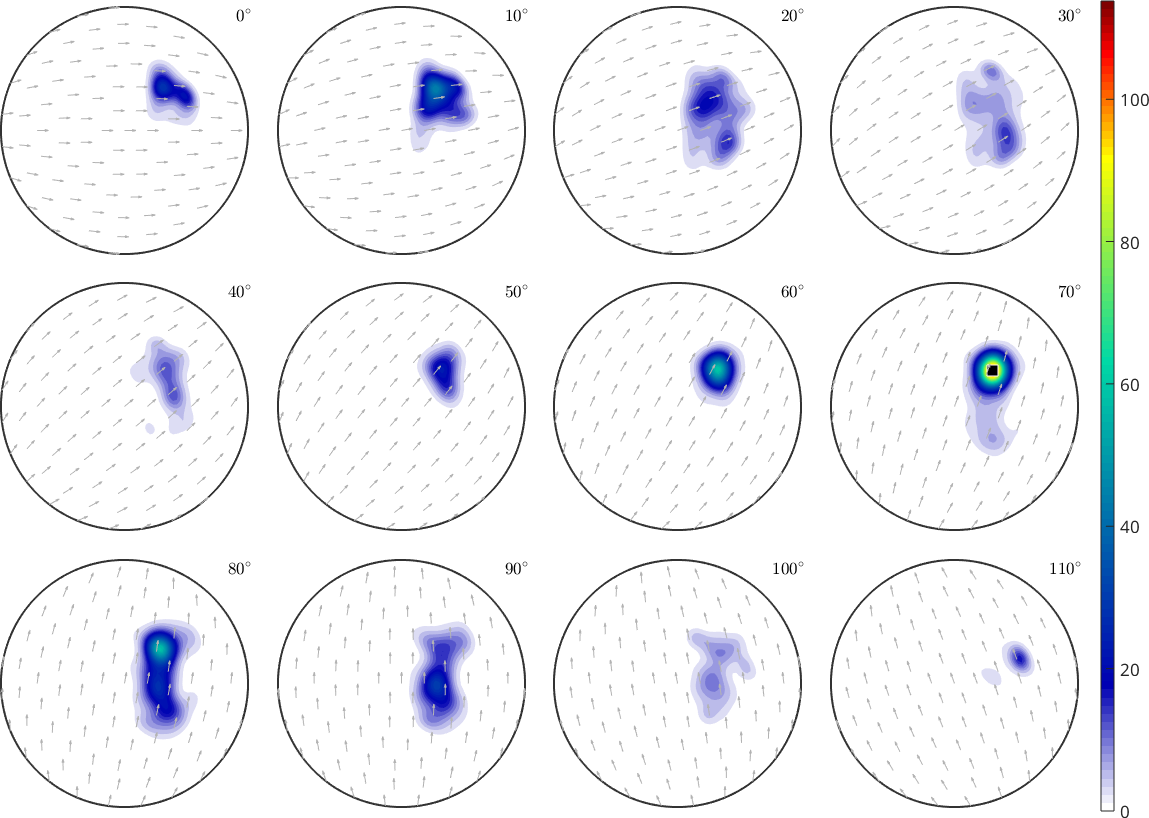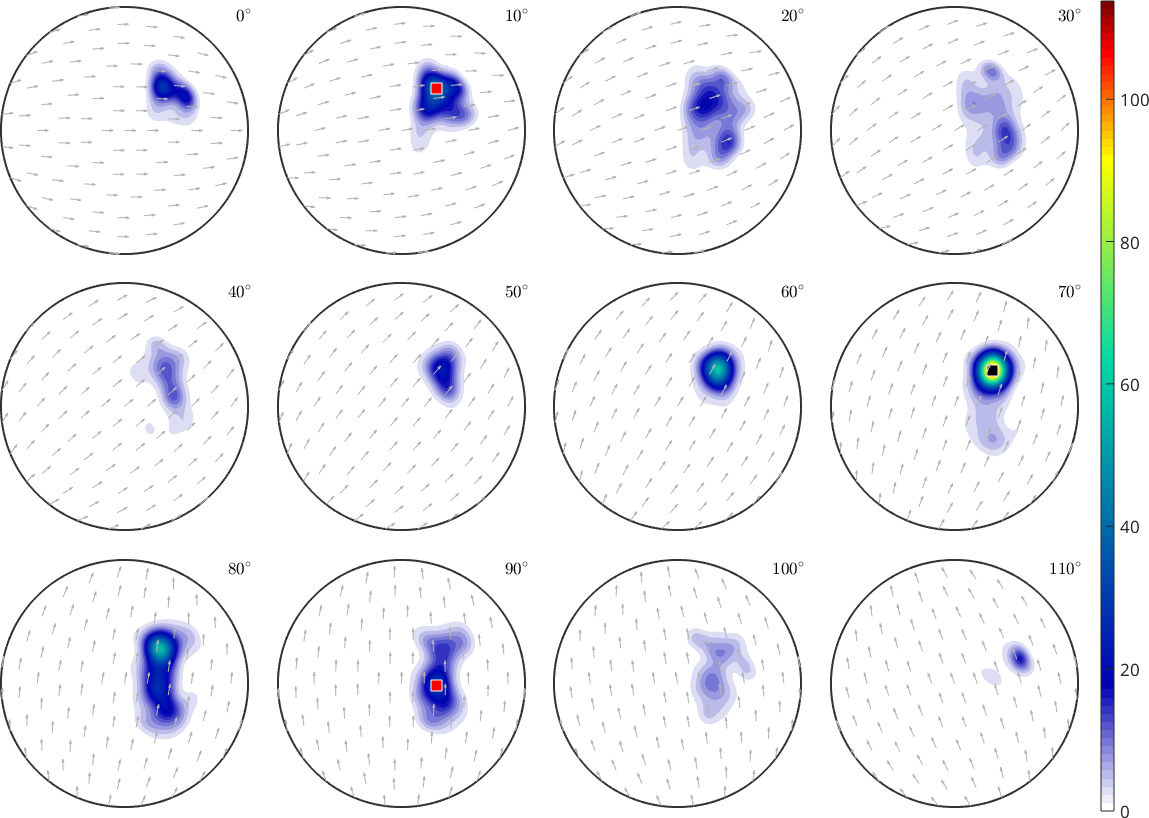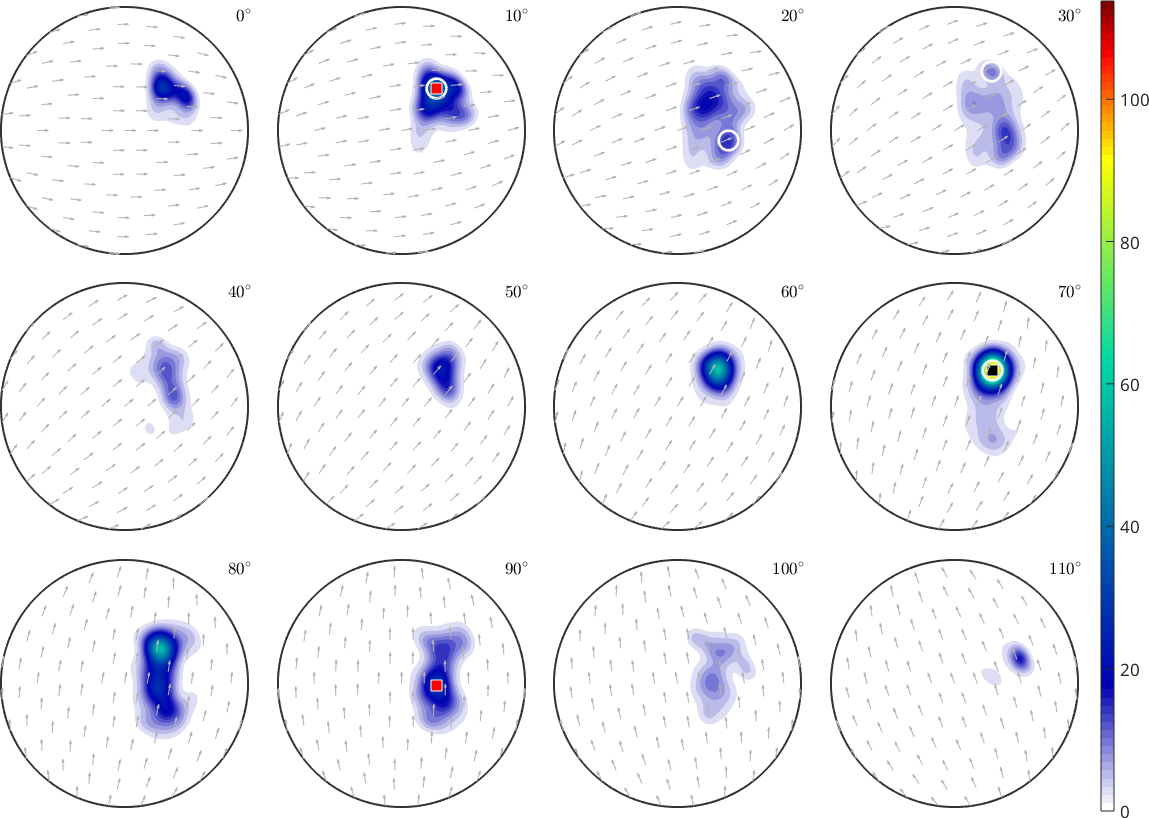ODF Component Analysis edit page

A common way to interprete ODFs is to think of them as superposition of different components that originate from different deformation processes and describe the texture of the material. In this section we describe how these components can be identified from a given ODF.

We start by reconstruction a Quarz ODF from Neutron pole figure data.## The prefered orientation

First of all we observe that the ODF posses a strong maximum. To find this orientation that correspondes to the maximum ODF intensity we use the max command.

Note that, similarly as the Matlab max command, the second output argument is the position where the maximum is atained. In our case we observe that the maximum value is about 121. To visualize the corresponding preferred orientation we plot it into the sigma sections of the ODF.We may not only use the command max to find the global maximum of an ODF but also to find a certain amount of local maxima. The number of local maxima MTEX should search for, is specified as by the option 'numLocal', i.e., to find the three largest local maxima doNote, that orientations are returned sorted according to their ODF value.

## Volume Portions

It is important to understand, that the value of the ODF at a preferred orientation is in general not sufficient to judge the importance of a component. Very sharp components may result in extremely large ODF values that represent only very little volume. A more robust and physically more relevant quantity is the relative volume of crystal that have an orientation close to the preferred orientation. This volume portion can be computed by the command volume(odf,ori,delta) where ori is a list of preferred orientations and delta is the maximum disorientation angle. Multiplying with $$100$$ the output will be in percent

We observe that the sum of all volume portions is far from $$100$$ percent. This is very typical. The reason is that the portion of the full orientations space that is within the $$10$$ degree disorientation distance from the preferred orientations is very small. More precisely, it represents only

percent of the entiere orientations space. Putting these values in relation it becomes clear, that all the components are multiple times stronger than the uniform distribution. We may compute these factors by

It is important to understand, that all these values above depend significantly from the chosen disorientation angle delta. If delta is chosen too large

it may even happen that the components overlap and the sum of the volumes exceeds 100 percent.

## Non circular components

A disadvantage of the approach above is that one is restricted to circular components with a fixed disorientation angle which makes it hard to analyze components that are close together. In such settings one may want to use the command calcComponents. This command starts with evenly distributed orientations and lets the crawl towards the closest prefered orientation. At the end of this process the command returns these prefered orientation and the percentage of orientations that crawled to each of them.

These volumes allways sums up to apprximately 100 percent. While the prefered orientations should be the same as those computed by the max command.